# GRE Subject Test: Math : Trapezoidal Rule

## Example Questions

### Example Question #1 : Numerical Integration

Solve the integral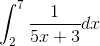using the trapezoidal approximation with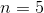subintervals.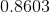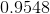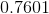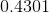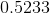Explanation:

Trapezoidal approximations are solved using the formula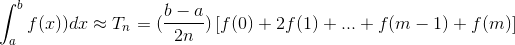whereis the number of subintervals and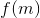is the function evaluated at the midpoint.

For this problem,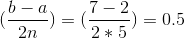.

The value of each approximation term is below.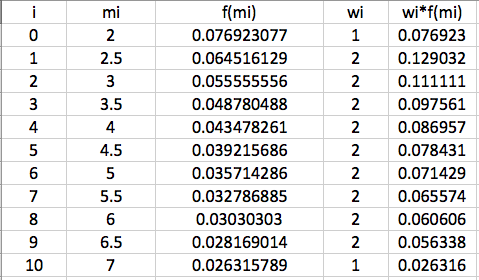The sum of all the approximation terms is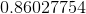, therefore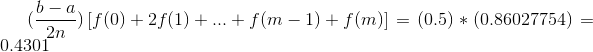### Example Question #11 : Differential Functions

Solve the integral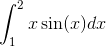using the trapezoidal approximation withsubintervals.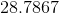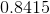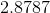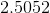Explanation:

Trapezoidal approximations are solved using the formulawhereis the number of subintervals andis the function evaluated at the midpoint.

For this problem,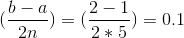.

The value of each approximation term is below.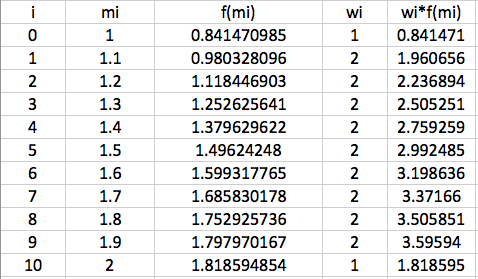The sum of all the approximation terms is, therefore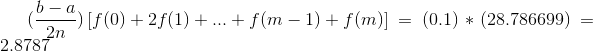### Example Question #1 : Trapezoidal Rule

Solve the integral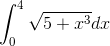using the trapezoidal approximation withsubintervals.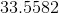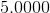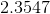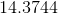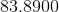Explanation:

Trapezoidal approximations are solved using the formula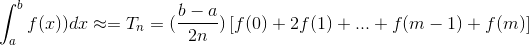whereis the number of subintervals andis the function evaluated at the midpoint.

For this problem,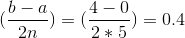.

The value of each approximation term is below.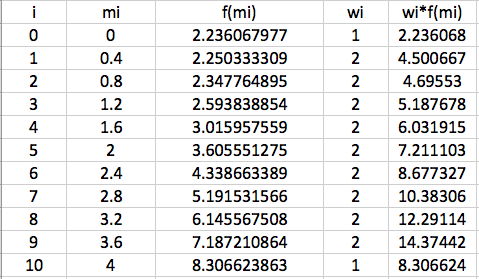The sum of all the approximation terms is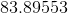, therefore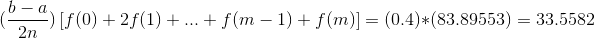### Example Question #2 : Trapezoidal Rule

Solve the integral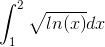using the trapezoidal approximation withsubintervals.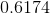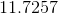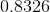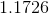Explanation:

Trapezoidal approximations are solved using the formulawhereis the number of subintervals andis the function evaluated at the midpoint.

For this problem,.

The value of each approximation term is below.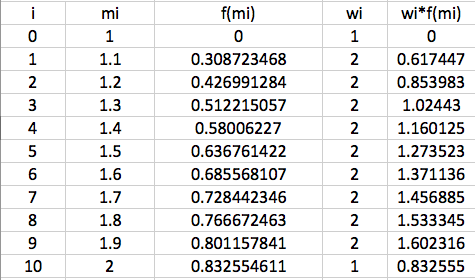The sum of all the approximation terms is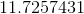, therefore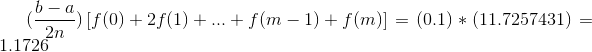### Example Question #1 : Numerical Integration

Evaluate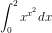using the Trapezoidal Rule, with n = 2.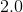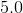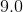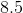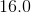Explanation:

1) n = 2 indicates 2 equal subdivisions. In this case, they are from 0 to 1, and from 1 to 2.

2) Trapezoidal Rule is: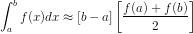3) For n = 2: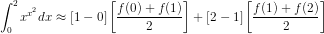4) Simplifying: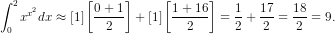### All GRE Subject Test: Math Resources